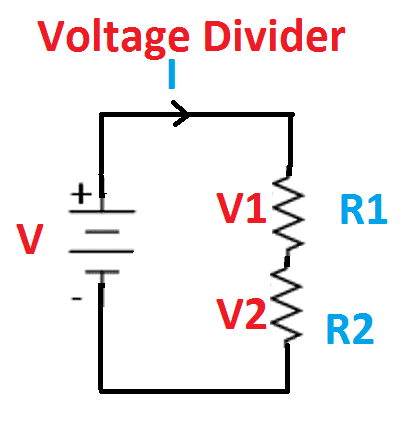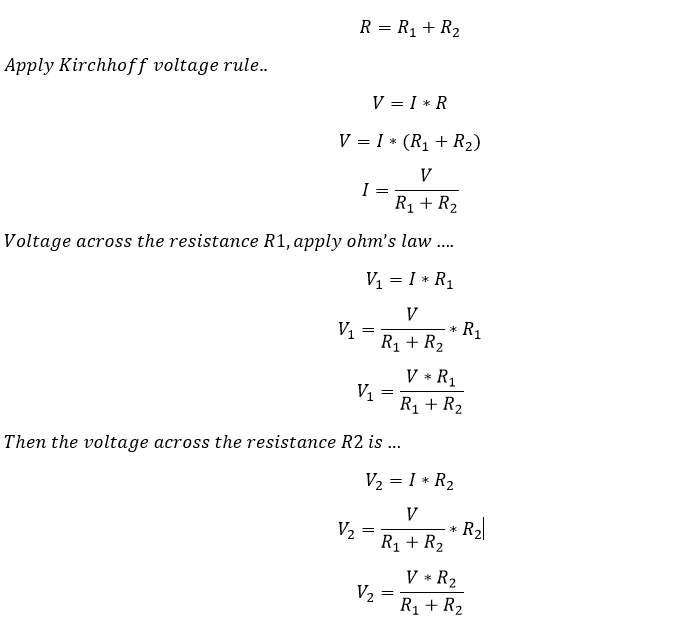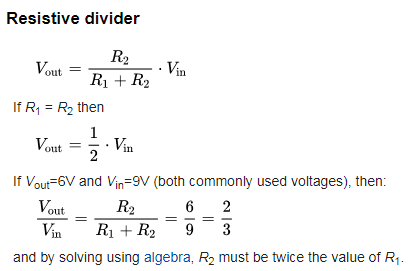# Voltage Divider or Potential divider:

Another important concept in the electrical circuit is Voltage divider rule. Voltage divider rule is generally used to find out the unknown voltage drop across the particular resistor. Consider a series circuit….Here the total resistance is equal to sum of the individual resistance of the circuit.Hence the voltage across the particular resistance is equal to source voltage times of the ratio between particular resistance to total series resistance of the circuit.

In such that, a series circuit can be called as voltage divider, this method is used mainly in electronics circuit designing. In electronics, a voltage divider (also known as a potential divider) is a passive linear circuit that produces an output voltage (Vout) that is a fraction of its input voltage (Vin). To provide accurate voltage, these divider circuits are used. For connecting n number of resistor in series then the voltage drop across each resistor can be expressed as..

Learn More:   Why Aluminium and Copper Vessels & Pot not Used in Induction Stove

Example:Here the R total is the sum of all series connected resistance.

Note: To apply a voltage divider rule, the circuit should be in series.

Current divider rule is used parallel circuit. The formula describing a voltage divider is similar in form to that for the current divider.

## Application of Voltage divider or Potential divider:

### Sensor measurement:

Voltage dividers can be used to allow a microcontroller to measure the resistance of a sensor. The sensor is wired in series with a known resistance to form a voltage divider, the known voltage is applied across the circuit. In this you know the Voltage drop across the resistance and sensor, at the same time microcontroller measure the resistance of the sensor.

### High voltage measurement:

A voltage divider can be used to measure high voltage. The high voltage is applied across the divider, and the divider output—the divider is used to reduce the input voltage with in the meter range. Finally, the voltage can be measured.

Learn More:   Horsepower Hp to Amps (hp to A) Conversion Calculator DC, 1 Phase, 3 Phase

### Logic level shifting:

A voltage divider can be used as a crude logic level shifter to interface two circuits that use different operating voltages.

### Potential divider:

By replacing a variable resistance by any of the resistance above-mentioned diagram, you can use them as a potential divider. You can vary the output voltage by varying the resistance value.